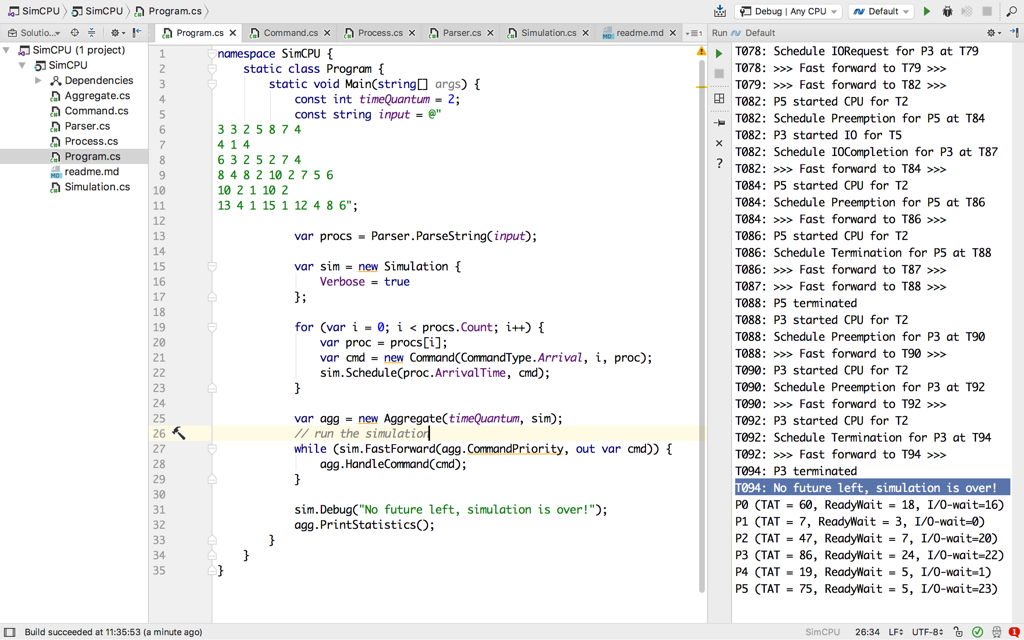# Simulate CPU Scheduler

This is a reference project for simulating event-driven systems and understanding the passing of time. It is a part of the materials for two R&D projects:

1. Deterministic simulation of event-driven systems (see High Availability and Performance).
2. Event-driven view engine (or "event-driven mainframe").

Source code is available on github.## Domain

Domain is based on the assignment: CS452 Project Job Scheduling Simulation.

The task is to create a Round-Robin job scheduler for the system with one CPU and one IO-device. This isn't going to be a real job scheduler, but rather a simulation.

• processes are scheduled to arrive at a specific time based on the spec;
• each process has a sequence of CPU and IO tasks with varying duration;
• process always ends with a CPU task;
• the system has only one CPU and one IO device, for which the processes compete;
• if a process needs more CPU time than `TimeQuantum`, then the scheduler can interrupt its execution and put back into the queue, allowing the other processes to move forward;
• if multiple commands are to happen at the same point in time, then the order is: (1) handle new process; (2) handle process coming back from IO queue and (3) handle preempted (interrupted) process.

## Implementation

In this implementation we explicitly model (spell out) following concepts:

A mixed workload with the `TimeQuantum` of 2 will be executed when you compile and run the project:

``````3 3 2 5 8 7 4
4 1 4
6 3 2 5 2 7 4
8 4 8 2 10 2 7 5 6
10 2 1 10 2
13 4 1 15 1 12 4 8 6
``````

Each line defines a single process. For instance, first line is:

• `3` - process arrival time (always ascending);
• `3` - number of CPU bursts;
• `2 5 8 7 4` - CPU for 2 time units, IO for 5... CPU for 4 time units.

## Pay attention

Time is an illusion, modeled by running the simulation towards the discrete commands we have planned in the future.

Future is represented as a list of scheduled commands sorted by their planned execution time.

Multiple commands could be scheduled at a single point in time. Domain logic (`Aggregate.CommandPriority` in this case) determines the order of command execution.

While handling a command, the domain logic could schedule another command to happen in the future.

The simulation ends when it has no future left.

## Questions

• What is a fuzz testing? How could it be applied to this project?
• How would you reproduce and debug issues discovered by the fuzz testing?

## Bonus Points

The implementation almost works. For some reason, while running the mixed job workload with `TimeQuantum=2`:

the expected result (according to the CS452) is:

``````P0 (TAT = 60, ReadyWait = 18, I/O-wait=16)
P1 (TAT = 7, ReadyWait = 3, I/O-wait=0)
P2 (TAT = 47, ReadyWait = 7, I/O-wait=20)
P3 (TAT = 86, ReadyWait = 24, I/O-wait=22)
P4 (TAT = 19, ReadyWait = 5, I/O-wait=1)
P5 (TAT = 75, ReadyWait = 7, I/O-wait=21)
``````

while the simulation actually returns:

``````P0 (TAT = 60, ReadyWait = 18, I/O-wait=16)
P1 (TAT = 7, ReadyWait = 3, I/O-wait=0)
P2 (TAT = 47, ReadyWait = 7, I/O-wait=20)
P3 (TAT = 86, ReadyWait = 24, I/O-wait=22)
P4 (TAT = 19, ReadyWait = 5, I/O-wait=1)
P5 (TAT = 75, ReadyWait = 5, I/O-wait=23)
``````

Note the different `ReadyWait` and `I/O-wait` values for `P5`.

Next post in Simulation story: Simulate Ring of Actors